Zero-Inflated Count Regression Overview

The main motivation for zero-inflated count models is that real-life data frequently display overdispersion and excess zeros. Zero-inflated count models provide a way of modeling the excess zeros in addition to allowing for overdispersion. In particular, for each observation, there are two possible data generation processes. The result of a Bernoulli trial is used to determine which of the two processes is used. For observation, Process 1 is chosen with probability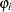and Process 2 with probability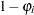. Process 1 generates only zero counts. Process 2 generates counts from either a Poisson or a negative binomial model. In general,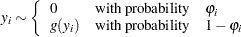Therefore, the probability of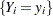can be described as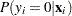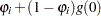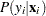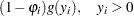where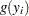follows either the Poisson or the negative binomial distribution. You can specify the probability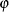with the PROBZERO= option in the OUTPUT statement.

When the probabilitydepends on the characteristics of observation,is written as a function of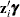, where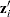is the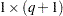vector of zero-inflation covariates and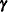is the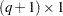vector of zero-inflation coefficients to be estimated. (The zero-inflation intercept is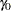; the coefficients for the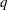zero-inflation covariates are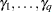.) The functionthat relates the product(which is a scalar) to the probabilityis called the zero-inflation link function,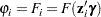In the TCOUNTREG procedure, the zero-inflation covariates are indicated in the ZEROMODEL statement. Furthermore, the zero-inflation link functioncan be specified as either the logistic function,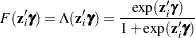or the standard normal cumulative distribution function (also called the probit function),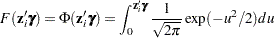The zero-inflation link function is indicated in the LINK option in ZEROMODEL statement. The default ZI link function is the logistic function.

Note: This procedure is experimental.